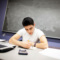# How To Write Math Research Papers, Term Papers & ThesisMost research papers and proofs in mathematics are written in CSE Style which is the official citation method of the Council of Science Editors as well as the American Math Society. Even so, the style can vary upon the type of paper as well as its length.

Mathematics refers to the academic discipline which centers upon concepts like quantity, structure, space as well as change. It refers to the science drawing necessary conclusions and also the science that looks for patterns in numbers, spaces and imaginary abstractions.

By using abstraction as well as logical reasoning, the subject has evolved using counting, measurement, calculation, and the shapes as well as motions of physical objects. Today, mathematics courses are used in most disciplines and mathematics courses are a part of college curriculums around the world in most majors. This includes science, engineering, social sciences and medicine.

Good mathematical writing is essential to expressing the thoughts and nature of a problem to the readers. The basic aim of almost all writing is to communicate. It is important to keep in mind what it is that needs to be communicated and to whom it is being communicated to. The essential purpose of writing mathematical papers is to state the truth in a mathematical statement with the use of logical deductions. The most important thing is to prove that the work is well-founded. This happens to be our first major goal when writing a mathematical paper.

A successful mathematical paper provides its readers 2 logical maps. The first is used to display the connection between the paper and the general mathematical knowledge out there. The other is used to provide readers with an internal logical structure of the work done.

The very first step towards a good paper is the statement of the theorem. Once the basic outline of the paper has been prepared, other aspects such as the formal or logical structure based upon definitions, theorems, as well as proofs are included.

When writing a proof, you have to put down a linear order, set of hypotheses as well as deductions that may not be linear in form.

A mathematical problem is a question or problem with a mathematical nature. A mathematical problem can be a real-world problem in which the principles of mathematics allows for a deep insight into the nature of an issue. At the same time a mathematical problem can be one of an abstract nature such as Hilbert's problems or a problem that refers to the nature of the subject itself like Russell's Paradox.

Typically, the first step for solving a problem is to make a mathematical model that relates to the problem. This can imply abstraction from the problem’s details and being careful not to miss out any specific details when converting a real world problem into a mathematical one. Once the solution to the mathematical problem is found, it is translated back into the original one. The subject involves the search for fundamental truths with respect to pattern, quantity, as well as change.

How To Write Math Research Papers, Term Papers & Thesis
0 Opinion